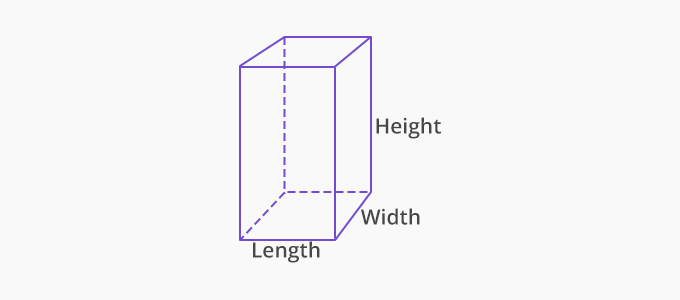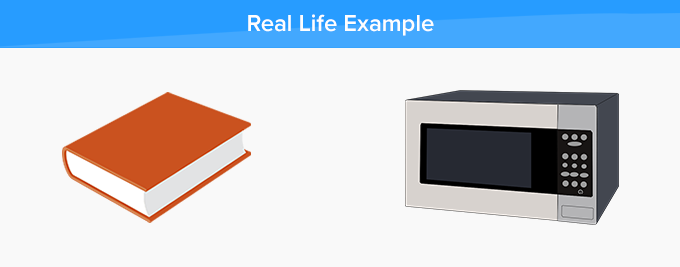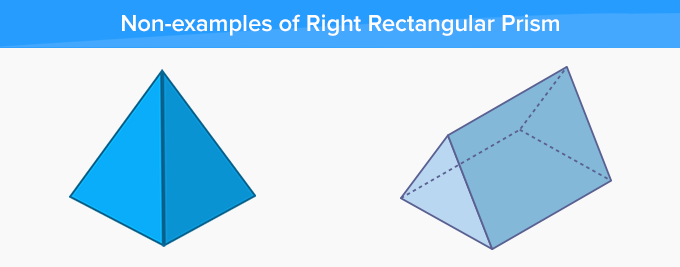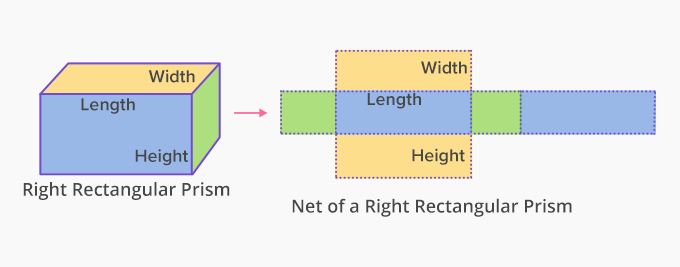# Right rectangular prism - Definition with Examples

The Complete Prek-5 Learning Program Built for Your Child
Home > Right rectangular prism

What is Right Rectangular Prism?

A right rectangular prism is a three-dimensional object with 6 faces, 12 edges and 8 vertices.In a right rectangular prism:

• the angles between the base and sides are right angles.

• all the 6 faces are rectangles.

This prism is also called a cuboid and each corner of the prism is a right angle. Each base and top of a rectangular prism is congruent; that is; exactly of the same shape and size.

Real life examples of a right rectangular prism:

Right rectangular prisms or cuboids are all around us. A few of the examples are books, boxes, buildings, bricks, boards, doors, containers, cabinets, mobiles, and laptops.Non-examples of right rectangular prism:

• This shape is a prism but its top and base do not have right angles in the shape. This is not a right rectangular prism.

• This shape is not a prism. Neither it has rectangles in  top and bottom nor there are any right angles in them. This is also not a right rectangular prism.Net of a right rectangular prism:

The net of a 3D object shows the faces of that object when it is opened flat. We can form a right rectangular prism using its net as shown below as each face of the net is a rectangle which has right angles into it.Fun Facts Rectangular prisms can have more than one net. A cube is a also a right rectangular prism with all its sides of equal length. If the bases of a right angle prism are square, then it is called a square prism.